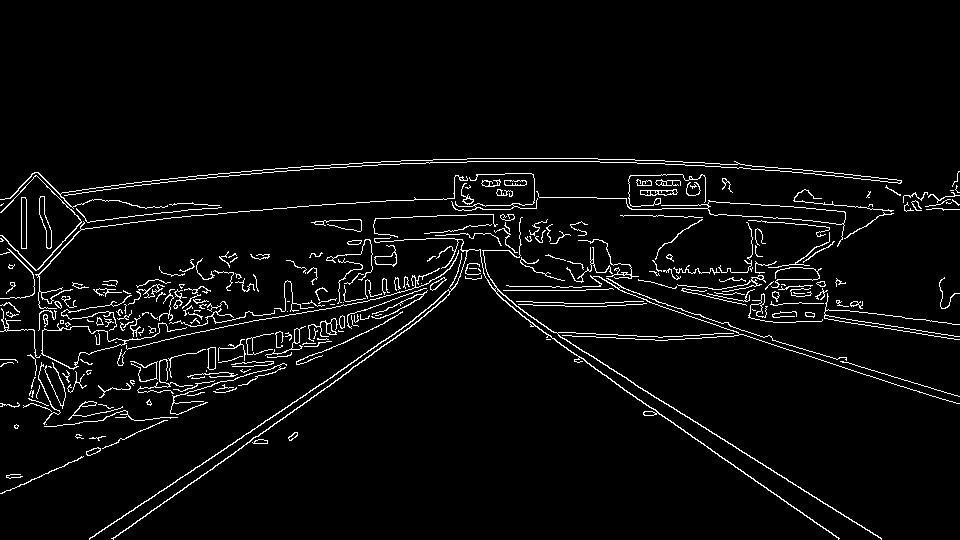#2019 iT 邦幫忙鐵人賽DAY 3
0
AI & Data

# 實作說明

## 讀取圖檔

``````import matplotlib.pyplot as plt
import matplotlib.image as mpimg
plt.imshow(image)
``````## 圖檔轉灰階

``````import cv2  #bringing in OpenCV libraries
gray = cv2.cvtColor(image, cv2.COLOR_RGB2GRAY) #grayscale conversion
plt.imshow(gray, cmap='gray')
``````## Canny邊緣檢測(Canny edge detector)應用說明

``````edges = cv2.Canny(gray, low_threshold, high_threshold)
``````

# 程式處理步驟

## 1.載入所需之程式庫

``````#doing all the relevant imports
import matplotlib.pyplot as plt
import matplotlib.image as mpimg
import numpy as np
import cv2
``````

## 2.讀取圖檔並轉成灰階

``````image = mpimg.imread('exit-ramp.jpg')
gray = cv2.cvtColor(image, cv2.COLOR_RGB2GRAY)
``````

## 3.為高斯平滑/模糊定義核心大小

``````kernel_size = 3
blur_gray = cv2.GaussianBlur(gray,(kernel_size, kernel_size), 0)
``````

## 4.調整Canny之參數

``````low_threshold = 1
high_threshold = 10
edges = cv2.Canny(blur_gray, low_threshold, high_threshold)
``````

## 5.顯示成果圖片

``````plt.imshow(edges, cmap='Greys_r')
``````